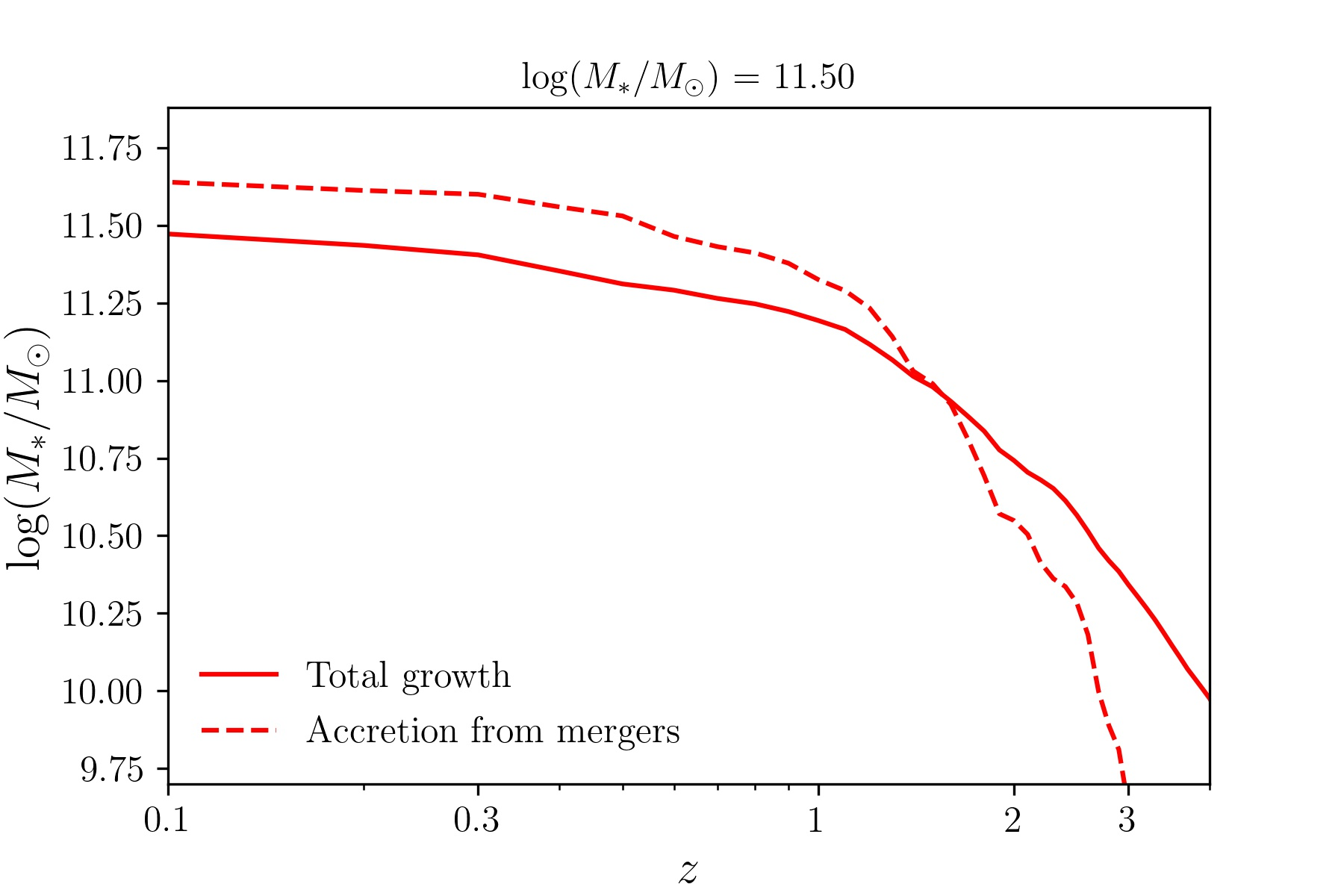### Average Merger Histories IssuesMax Dickson
• 2
• 10 Mar

I am writing a function to extract the average merger histories of galaxies.

My method is as follows:

2) take the main leaf progenitor of the root halo at redshift = 0 to get the index
3) using the halo index, take the next progenitor
4) calculate the stellar mass of the halo before accretion using the same method as in sublink.maxPastMass and the snapshot when masses = np.max(masses)
5) append both the mass and snapshot to arrays
6) loop from step 3 until npID = -1
7) take the next descendent to use as the next index
8) loop from step 3 until next descendent ID = -1
9) at each snapshot add up the masses of the galaxies accreted
10) cumulatively add up the masses from snapshot = 0 to snapshot = 99

When I compare the result of this method to the total growth (from total_growth = tree['SubhaloMassInRadType'][:,4], where tree = sublink.loadTree), I end up with the mass contribution from mergers exceeding the total growth, as shown for the average of galaxies where log(M_star/M_sun) = 11.5 below:Any help would be greatly appreciated!Dylan Nelson
• 10 Mar

Hi,

First, physically, I imagine that for a given halo the total cumulative mass growth through mergers could be smaller than, equal to, or larger than the actual mass growth. If merger material eventually leaves (e.g. stripping), then it would be larger. If significant "smooth" accretion also takes place, then it could be smaller.

That said, I think the definition of (4) is critical here. You should think more about, if this definition of "previous maximum past mass" makes sense for your calculation?Max Dickson
• 10 Mar

Yes, you are most likely right that step 4 is the issue. To be clear, I wasn't expecting the merger contribution to be the exact same as the total growth as there are other processes that contribute to the parent galaxies growth. Is there perhaps a method that you are aware of that could isolate the stellar mass accreted strictly from mergers that could replace step 4?Dylan Nelson
• 10 Mar

Perhaps looking at the methods used to derive e.g. `StellarMassExSitu` would be useful, in the Stellar Assembly post-processing catalog (and paper references).

• Page 1 of 1